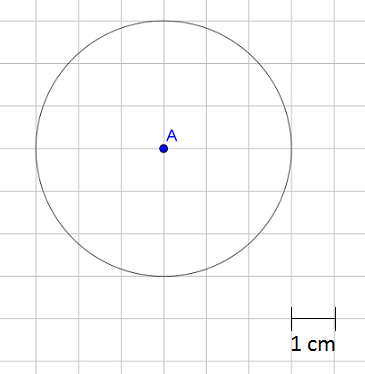# Geometry

## Objective

Determine the relationship between the area and radius of a circle and use it to solve problems.

## Common Core Standards

### Core Standards

?

• 7.G.B.4 — Know the formulas for the area and circumference of a circle and use them to solve problems; give an informal derivation of the relationship between the circumference and area of a circle.

## Criteria for Success

?

1. Approximate the area of a circle using a grid.
2. Informally derive the formula for the area of a circle using wedges of a circle.
3. Know the formula that relates the area and circumference of a circle: ${{A=\pi r^2}}$.
4. Use the formula ${{A=\pi r^2}}$ to solve problems.

## Tips for Teachers

?

Lessons 8 and 9 focus on the relationship between a circle’s area and its radius. In Lesson 8, students determine this relationship and the formula that represents it. In Lesson 9, students will solve real-world and mathematical problems involving area.

#### Remote Learning Guidance

If you need to adapt or shorten this lesson for remote learning, we suggest prioritizing Anchor Problem 2 (benefits from discussion) and Anchor Problem 3 (benefits from worked example). Students can use the interactive Academo applet (linked to the right) to explore finding the area of a circle and then discuss As a discovery problem, if live discussion or reflection of the problem were possible, it would allow for students to arrive at the conclusion on their own. Find more guidance on adapting our math curriculum for remote learning here.

#### Fishtank Plus

• Problem Set
• Student Handout Editor
• Vocabulary Package

## Anchor Problems

?

### Problem 1

Use the centimeter grid to find an approximate area of the circle. Explain why your approximation is reasonable.### Problem 2

Use this applet from Academo, Area of a circle (Rearrangement Method) to informally derive the relationship between the area of a circle and its circumference

Start with a small number of sectors or wedges of the circle and then slowly increase the number. Click “rearrange” after each number. Ask:

• How are the circle wedges being rearranged?
• What shape are the rearranged pieces taking?
• What are the dimensions of the rearranged shape?
• How can you determine the area of a circle?

### Problem 3

Use the relationship between area and radius to solve the two problems below.

1. The radius of a circular patch of grass is 3 yards. What is the area of the patch of grass?
2. The area of a circular plate is approximately ${50.24 \space\mathrm{in^2}}$. What is the radius of the plate?

## Problem Set

?The following resources include problems and activities aligned to the objective of the lesson that can be used to create your own problem set.

• Include spiraled review from the unit, including procedural problems solving equations if additional practice is needed.

?

### Problem 1

A circle is divided into 16 equal wedges, as shown below. Explain or show how you can rearrange the pieces to determine the area of the circle.### Problem 2

Find the area of a circle that has a radius of 5 inches.

?